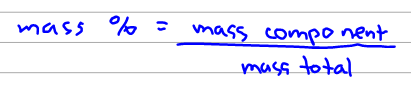# Problem: Calculate the percentage by mass of oxygen in the following compounds.codeine, C18H21NO3

🤓 Based on our data, we think this question is relevant for Professor Bartoszek Loza's class at OSU.

###### FREE Expert Solution

The mass percent can be calculated using the formula below:Before we can solve the mass %, we have to calculate mass of codeine and mass of oxygen.###### Problem Details

Calculate the percentage by mass of oxygen in the following compounds.

codeine, C18H21NO3